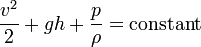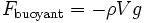# Wind / Fluid Dynamics.

## Homework Statement

A sheet of aluminum of thickness .01 m lies in a parking lot. A wind blows over the top of the sheet. What is the minimum velocity that will cause the sheet to begin to rise: Assume the density of air is 1.18 kg/m^3 and aluminum is 2700 kg/m^3.

## Homework Equations

Bernoulli's Equation:or

Archimedes Principal:?[/URL]

Density = M/Vol

## The Attempt at a Solution

I really have no idea where to start. Since the density of AL is different than that of the fluid, I would assume it to be a buoyant force. However, air is a strange fluid to work with, and I can't figure out the volume displaced.

I just need a hint on where to begin... maybe some links to other reading. All help would be vastly appreciated.

Thanks! Sean

Last edited by a moderator: